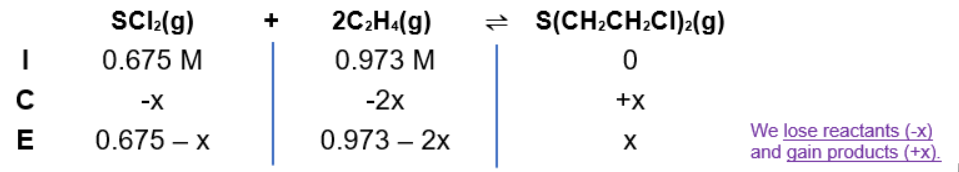# Problem: A toxicologist studying mustard gas, S(CH2CH2Cl)2, a blistering agent, prepares a mixture of 0.675 M SCl2 and 0.973 M C2H4 and allows it to react at room temperature (20.0°C):SCl2(g) + 2C2H4(g) ⇌ S(CH2CH2Cl)2(g)At equilibrium, [S(CH2CH2Cl)2] = 0.350 M. Calculate Kp.

###### FREE Expert Solution

Equilibrium equation: SCl2(g) + 2C2H4(g) ⇌ S(CH2CH2Cl)2(g)

When dealing with equilibrium:

Kc → equilibrium units are in molarity
Kp → equilibrium units in terms of pressure

Kc is an equilibrium expression:

$\overline{){{\mathbf{K}}}_{{\mathbf{c}}}{\mathbf{=}}\frac{\mathbf{products}}{\mathbf{reactants}}{\mathbf{=}}\frac{\left[S{\left({\mathrm{CH}}_{2}{\mathrm{CH}}_{2}\mathrm{Cl}\right)}_{2}\right]}{\mathbf{\left[}{\mathbf{SCl}}_{\mathbf{2}}\mathbf{\right]}{\mathbf{\left[}{\mathbf{C}}_{\mathbf{2}}{\mathbf{H}}_{\mathbf{4}}\mathbf{\right]}}^{\mathbf{2}}}}$

Kp and Kc are related:

$\overline{){{\mathbf{K}}}_{{\mathbf{p}}}{\mathbf{=}}{{\mathbf{K}}}_{{\mathbf{c}}}{\left(\mathbf{RT}\right)}^{\mathbf{∆}\mathbf{n}}}$

ICE ChartAt equilibrium: [S(CH2CH2Cl)2] = x = 0.350 M

• [SCl2] = 0.675 - 0.350 = 0.325 M
• [C2H4] = 0.973 - 2(0.350) = 0.273 M

$\overline{){{\mathbf{K}}}_{{\mathbf{c}}}{\mathbf{=}}\frac{\left[S{\left({\mathrm{CH}}_{2}{\mathrm{CH}}_{2}\mathrm{Cl}\right)}_{2}\right]}{\mathbf{\left[}{\mathbf{SCl}}_{\mathbf{2}}\mathbf{\right]}{\mathbf{\left[}{\mathbf{C}}_{\mathbf{2}}{\mathbf{H}}_{\mathbf{4}}\mathbf{\right]}}^{\mathbf{2}}}}\phantom{\rule{0ex}{0ex}}{\mathbf{K}}_{\mathbf{c}}\mathbf{=}\frac{\left[\mathbf{0}\mathbf{.}\mathbf{350}\right]}{\left[\mathbf{0}\mathbf{.}\mathbf{325}\right]{\left[\mathbf{0}\mathbf{.}\mathbf{273}\right]}^{\mathbf{2}}}$

Kc = 14.45

91% (221 ratings)###### Problem Details

A toxicologist studying mustard gas, S(CH2CH2Cl)2, a blistering agent, prepares a mixture of 0.675 M SCl2 and 0.973 M C2H4 and allows it to react at room temperature (20.0°C):
SCl2(g) + 2C2H4(g) ⇌ S(CH2CH2Cl)2(g)
At equilibrium, [S(CH2CH2Cl)2] = 0.350 M. Calculate Kp.

Frequently Asked Questions

What scientific concept do you need to know in order to solve this problem?

Our tutors have indicated that to solve this problem you will need to apply the ICE Chart concept. You can view video lessons to learn ICE Chart. Or if you need more ICE Chart practice, you can also practice ICE Chart practice problems.

What professor is this problem relevant for?

Based on our data, we think this problem is relevant for Professor Gao's class at EKU.

What textbook is this problem found in?

Our data indicates that this problem or a close variation was asked in Chemistry: The Molecular Nature of Matter and Change - Silberberg 8th Edition. You can also practice Chemistry: The Molecular Nature of Matter and Change - Silberberg 8th Edition practice problems.